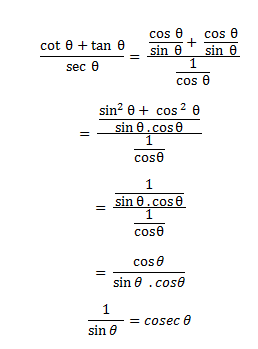# SSC CGL 2018 Practice Test Papers | Quantitative Aptitude (Day-62)

Dear Aspirants, Here we have given the Important SSC CGL Exam 2018 Practice Test Papers. Candidates those who are preparing for SSC CGL 2018 can practice these questions to get more confidence to Crack SSC CGL 2018 Examination.

[WpProQuiz 3786]

Click “Start Quiz” to attend these Questions and view Explanation

1) The sum of three consecutive natural numbers is always divisible by ____________

a) 2

b) 3

c) 4

d) 5

2) 3/4th of the tank filled with wine. After taking 60 litres on wine the tank is 2/3rd part full. What is the capacity of (in litres) of the tank?

a) 240

b) 360

c) 720

d) 600

3) Adding all natural numbers from 1 to 20, however one number is missed due to which the sum becomes 190. What is that missing number?

a) 9

b) 15

c) 10

d) 20

4) Kavita is thrice as efficient as Savita. Kavita can complete a work in 60 days less than Savita. In how man day the work will be completed if they work together?

a) 15

b) 22.5

c) 17.5

d) 10

5) If x – y : y – z : z – k = 2 : 3 : 5, then what is the value of z : (x + k)?

a) 1: 2

b) 1: 4

c) 1: 6

d) 1: 8

6) A shop keeper gains 25% profit while selling an article. If he buys half of the rate and sells same selling price, then what is his profit percentage?

a) 100%

b) 125%

c) 150%

d) 175%

7) If the side of the cube id increased by 10%, then what will be the percentage increased in the volume of cube?

a) 30

b) 30.1

c) 35

d) 33.1

8) What will be the amount on Rs 24000 at the rate of 35% per annum compounded yearly for 2 years?

a) 43750

b) 43740

c) 47340

d) 45670

9) What is the curved surface area (in cm2) of a cylinder having radius of base as 21 cm and height as 30 cm?

a) 4230

b) 4290

c) 3960

d) 3690

10) What is the value of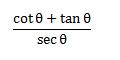a) Sec θ

b) Cosec θ

c) Cot θ

d) 1/ sec θ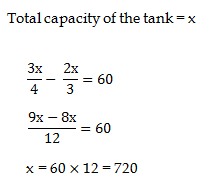Sum of All natural number from 1 to 20 = n (n+1)/2 = 20 * 21 /2 = 210

Missed number = 210 – 190 = 20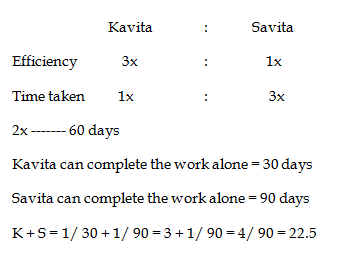x – y : y – z : z – k = 2 : 3 : 5

x – y = 2   ——— (1)

y – z = 3  ———- (2)

z – k = 5 ———- (3)

Let the value of k = 1

z – 1 = 5

z = 6 substitute the value in (2)

y – z = 3

y – 6 = 3

y = 9 substitute the value in (1)

x – y = 2

x – 9 = 2

x = 11

z : (x + k)

6 : (11 + 1)

6 : 12

1 : 2

Let the cost price = 100

Selling price = 125

If he bought half of the rate = 100 / 2 = 50

New cost price = 50

Same selling price = 125

Profit = 75

Profit % = (75 ×100/50) = 150%

7). Answer: d)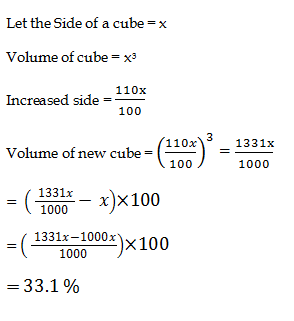Amount = Principal + Interest

A= P (1+r/100) ^n

A= 24000 (1 + 35/100)2

A = 24000 (135/100)2

A = 24000 (18225/10000)

A = 24 * 1822.5 =43740

Curved surface area of cylinder = 2πrh

= 2 × 22/ 7 × 21 × 30 = 2 ×22 ×3 ×30 = 44 × 90 = 3960